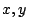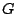# Possible Structure Theorem for Berge Graphs

Conjecture For every even-pair- free Berge graph, either it or its complement is the line graph of a bipartite graph, or has a 2-join.

A direct proof (if there is one) might give a shorter proof of the SPGC.

Contributed by Robin Thomas

A general even pair is not a composition- a non-Berge graph may become Berge by contracting an even pair.

How much more do we need in order to be able to find a construction for Berge graphs using even pairs?

Question Give a sufficient condition such that ifis an even pair satisfying the condition thenis Berge if and only if the graph obtained fromby contractingis Berge.

Contributed by Paul Seymour

Back to the main index for Perfect Graphs.